Next: Flow Past a Cylindrical Up: Two-Dimensional Incompressible Inviscid Flow Previous: Two-Dimensional Vortex Filaments

# Two-Dimensional Irrotational Flow in Cylindrical Coordinates

In a two-dimensional flow pattern, we can automatically satisfy the incompressibility constraint,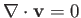, by expressing the pattern in terms of a stream function. Suppose, however, that, in addition to being incompressible, the flow pattern is also irrotational. In this case, Equation (5.10) yields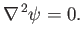(5.60)

In cylindrical coordinates, because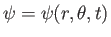, this expression implies that (see Section C.3)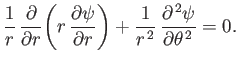(5.61)

Let us search for a separable solution of Equation (5.61) of the form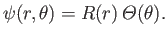(5.62)

It is easily seen that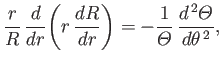(5.63)

which can only be satisfied if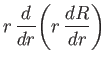(5.64)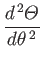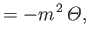(5.65)

where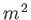is an arbitrary (positive) constant. The general solution of Equation (5.65) is a linear combination of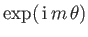and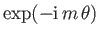factors. However, assuming that the flow extends over all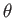values, the function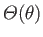must be single-valued in, otherwise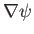--and, hence,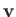--would not be be single-valued (which is unphysical). It follows thatcan only take integer values (and thatmust be a positive, rather than a negative, constant). The general solution of Equation (5.64) is a linear combination of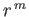and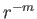factors, except for the special case, when it is a linear combination of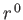and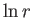factors. Thus, the general stream function for steady two-dimensional irrotational flow (that extends over all values of) takes the form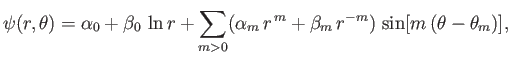(5.66)

where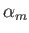,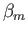, and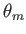are arbitrary constants. We can recognize the first few terms on the right-hand side of the previous expression. The constant termhas zero gradient, and, therefore, does not give rise to any flow. The term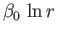is the flow pattern generated by a vortex filament of intensity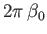, coincident with the-axis. (See Section 5.6.) The term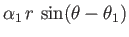corresponds to uniform flow of speed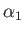whose direction subtends a (counter-clockwise) angle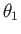with the minus-axis. (See Section 5.4.) Finally, the term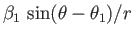corresponds to a dipole flow pattern. (See Section 5.5.)

The velocity potential associated with the irrotational stream function (5.66) satisfies [see Equations (4.89) and (5.7)]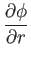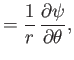(5.67)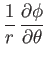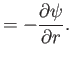(5.68)

It follows that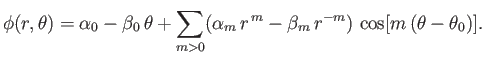(5.69)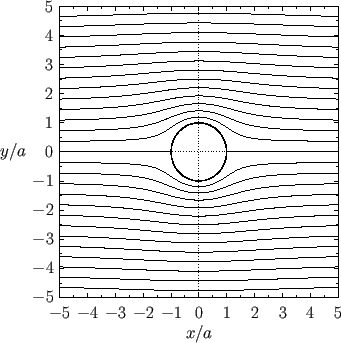Next: Flow Past a Cylindrical Up: Two-Dimensional Incompressible Inviscid Flow Previous: Two-Dimensional Vortex Filaments
Richard Fitzpatrick 2016-03-31